Ex 9.2

Chapter 9 Class 11 Sequences and Series
Serial order wise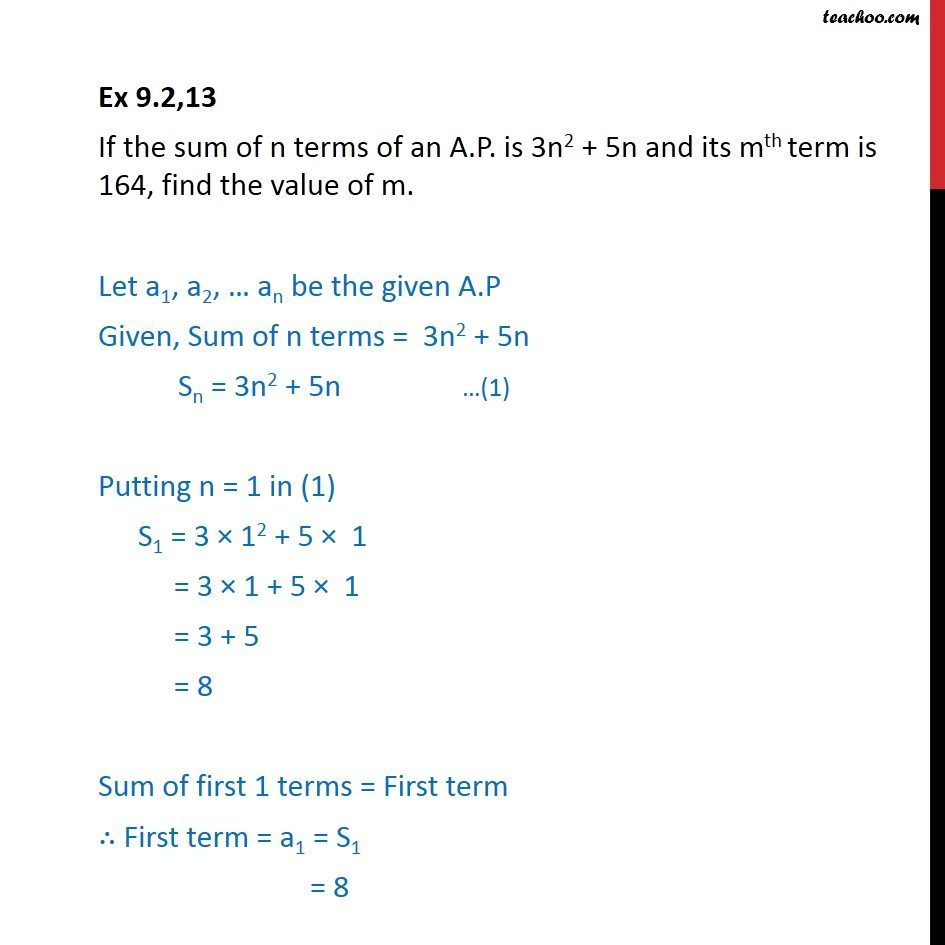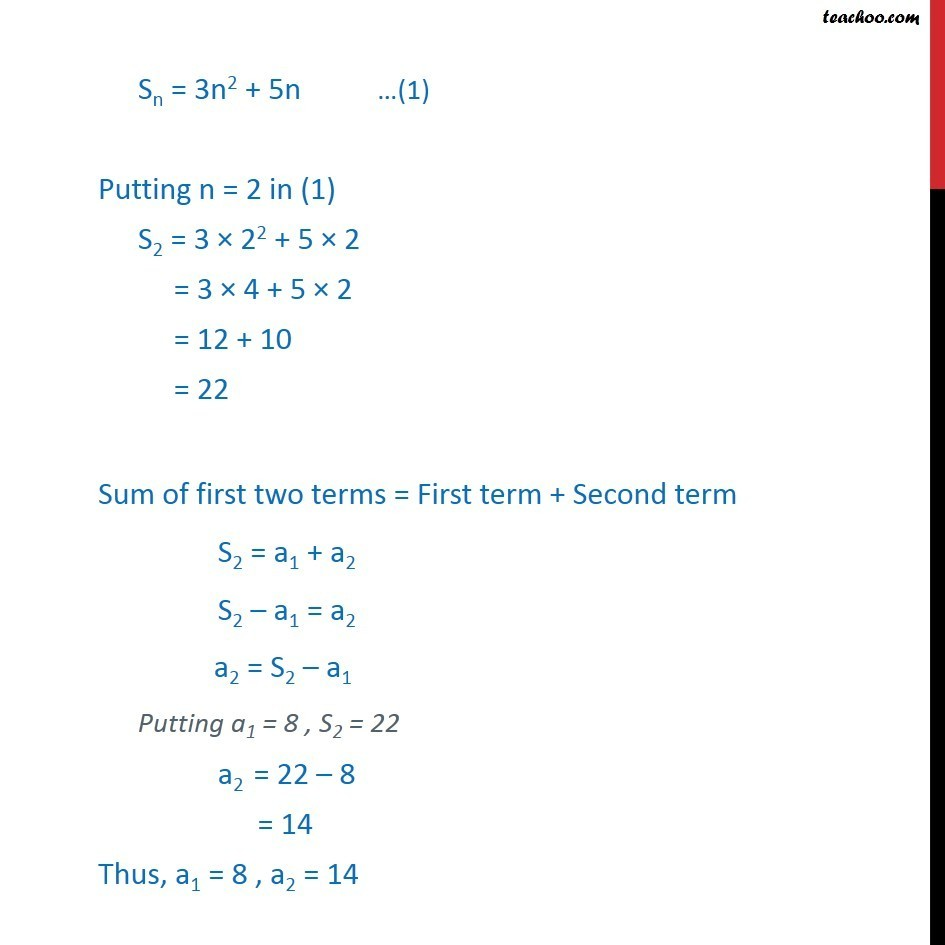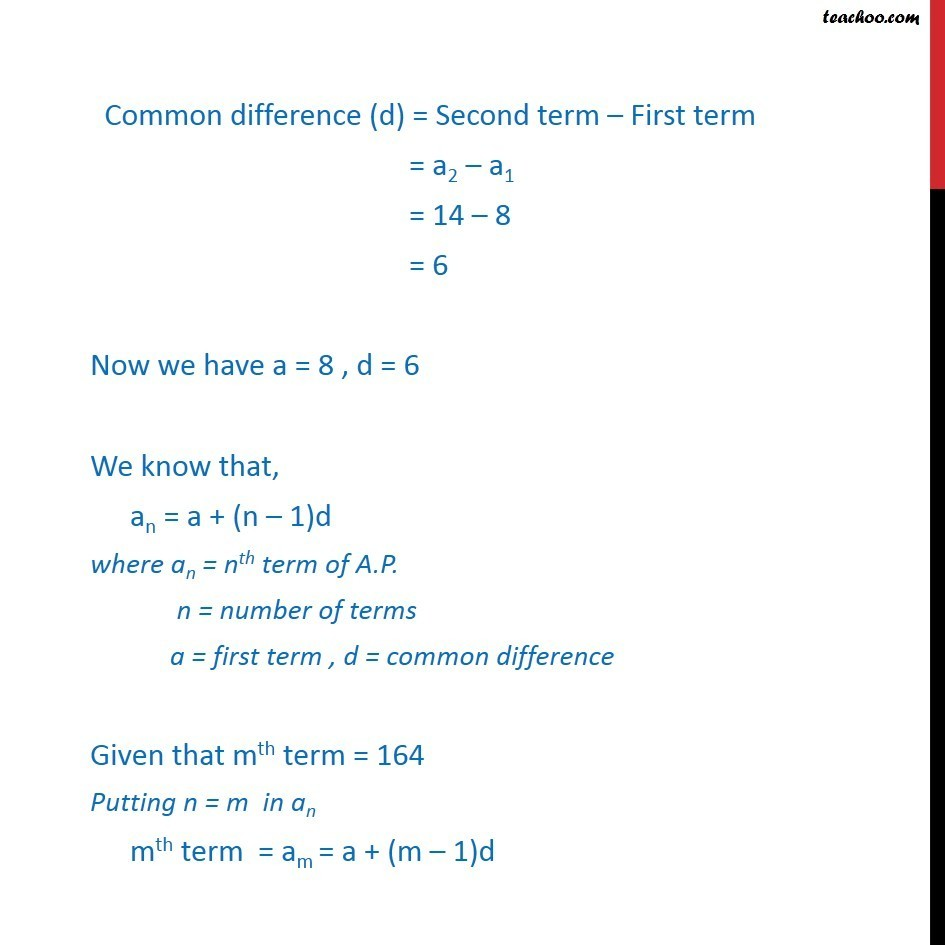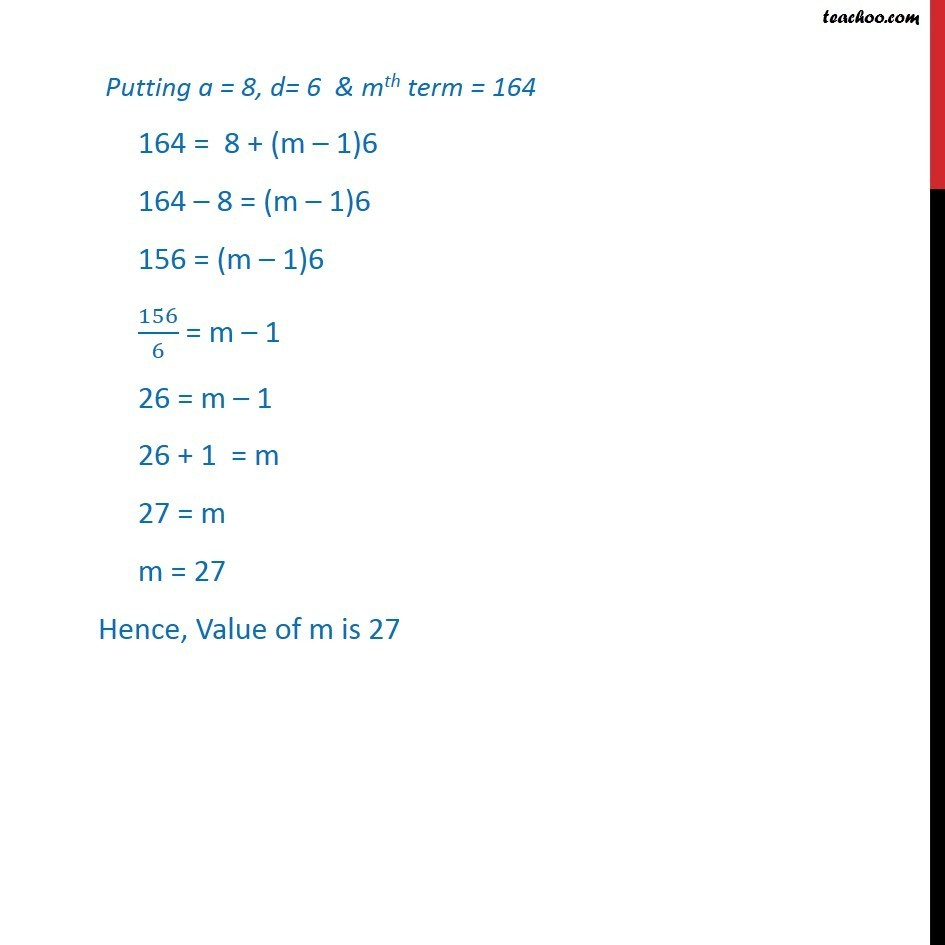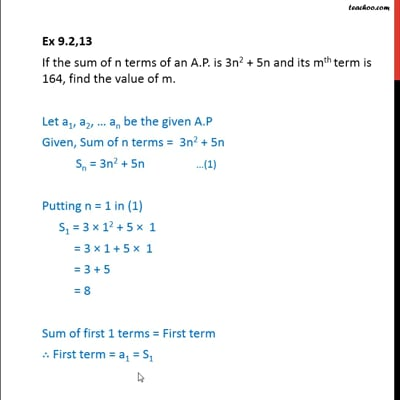This video is only available for Teachoo black users

Solve all your doubts with Teachoo Black (new monthly pack available now!)

### Transcript

Ex 9.2,13 If the sum of n terms of an A.P. is 3n2 + 5n and its mth term is 164, find the value of m. Let a1, a2, … an be the given A.P Given, Sum of n terms = 3n2 + 5n Sn = 3n2 + 5n Putting n = 1 in (1) S1 = 3 × 12 + 5 × 1 = 3 × 1 + 5 × 1 = 3 + 5 = 8 Sum of first 1 terms = First term ∴ First term = a1 = S1 = 8 Sn = 3n2 + 5n …(1) Putting n = 2 in (1) S2 = 3 × 22 + 5 × 2 = 3 × 4 + 5 × 2 = 12 + 10 = 22 Sum of first two terms = First term + Second term S2 = a1 + a2 S2 – a1 = a2 a2 = S2 – a1 Putting a1 = 8 , S2 = 22 a2 = 22 – 8 = 14 Thus, a1 = 8 , a2 = 14 Common difference (d) = Second term – First term = a2 – a1 = 14 – 8 = 6 Now we have a = 8 , d = 6 We know that, an = a + (n – 1)d where an = nth term of A.P. n = number of terms a = first term , d = common difference Given that mth term = 164 Putting n = m in an mth term = am = a + (m – 1)d Putting a = 8, d= 6 & mth term = 164 164 = 8 + (m – 1)6 164 – 8 = (m – 1)6 156 = (m – 1)6 156/6 = m – 1 26 = m – 1 26 + 1 = m 27 = m m = 27 Hence, Value of m is 27